## Example Questions

← Previous 1

### Example Question #1 : How To Find The Endpoints Of A Line Segment

The midpoint of line segment AB is (2, -5).  If the coordinates of point A are (4, 4), what are the coordinates of B?

(0, -13)

(6, 14)

(3, -0.5)

(6, 13)

(0, -14)

(0, -14)

Explanation:

The fastest way to find the missing endpoint is to determine the distance from the known endpoint to the midpoint and then performing the same transformation on the midpoint.  In this case, the x-coordinate moves from 4 to 2, or down by 2, so the new x-coordinate must be 2-2 = 0.  The y-coordinate moves from 4 to -5, or down by 9, so the new y-coordinate must be -5-9 = -14.

An alternate solution would be to substitute (4,4) for (x1,y1) and (2,-5) for (x,y) into the midpoint formula:

x=(x1+x2)/2

y=(y1+y2)/2

Solving each equation for (x2,y2) yields the solution (0,-14).

### Example Question #1 : How To Find The Endpoints Of A Line Segment

Point A is (5, 7).  Point B is (x, y).  The midpoint of AB is (17, –4). What is the value of B?

(12, –11)

(8.5, –2)

(22, –9)

(29, –15)

(29, –15)

Explanation:

Point A is (5, 7). Point B is (x, y). The midpoint of AB is (17, –4). What is the value of B?

We need to use our generalized midpoint formula:

MP = ( (5 + x)/2, (7 + y)/2 )

Solve each separately:

(5 + x)/2 = 17 → 5 + x = 34 → x = 29

(7 + y)/2 = –4 → 7 + y = –8 → y = –15

Therefore, B is (29, –15).

### Example Question #1 : How To Find The Endpoints Of A Line Segment

Line segment AB has an endpoint, A, located at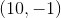, and a midpoint at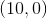. What are the coordinates for point B of segment AB?

The second endpoint cannot exist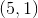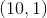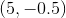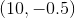Explanation:

With an endpoint A located at (10,-1), and a midpoint at (10,0), we want to add the length from A to the midpoint onto the other side of the segment to find point B. The total length of the segment must be twice the distance from A to the midpoint.

A is located exactly one unit below the midpoint along the y-axis, for a total displacement of (0,1). To find point B, we add (10+0, 0+1), and get the coordinates for B: (10,1).

### Example Question #4 : How To Find The Endpoints Of A Line Segment

Solve each problem and decide which is the best of the choices given.

What is the distance between the points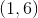and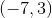on a standard coordinate plane?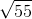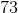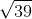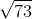Explanation:

Make a triangle. The points are 8 units apart on the-axis, andunits apart on the-axis. Then use the Pythagorean Theorem to find the distance of the hypotenuse, which ends up being.

Another way to solve this problem is to use the distance formula,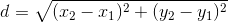Plugging in the two points we get,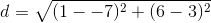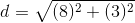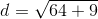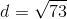### Example Question #1 : How To Find The Midpoint Of A Line Segment

A line segment has endpoints (0,4) and (5,6). What are the coordinates of the midpoint?

(3,9)

(2.5,-5)

(0,6)

(0,4)

(2.5,5)

(2.5,5)

Explanation:

A line segment has endpoints (0,4) and (5,6). To find the midpoint, use the midpoint formula:

X: (x1+x2)/2 = (0+5)/2 = 2.5

Y: (y1+y2)/2 = (4+6)/2 = 5

The coordinates of the midpoint are (2.5,5).

### Example Question #1 : How To Find The Midpoint Of A Line Segment

Find the midpoint between (-3,7) and (5,-9)

(-1,-1)

(4,-1)

(1,-1)

(4,-8)

(1,-8)

(1,-1)

Explanation:

You can find the midpoint of each coordinate by averaging them.  In other words, add the two x coordinates together and divide by 2 and add the two y coordinates together and divide by 2.

x-midpoint = (-3 + 5)/2 = 2/2 = 1

y-midpoint = (7 + -9)/2 = -2/2 = -1

(1,-1)

### Example Question #1 : How To Find The Midpoint Of A Line Segment

Find the coordinates for the midpoint of the line segment that spans from (1, 1) to (11, 11).

(5, 5)

(6, 6)

(7, 7)

(6, 5)

(5, 6)

(6, 6)

Explanation:

The correct answer is (6, 6). The midpoint formula is ((x+ x2)/2),((y+ y2)/2) So 1 + 11 = 12, and 12/2 = 6 for both the x and y coordinates.

### Example Question #1 : How To Find The Midpoint Of A Line Segment

What is the midpoint between the points (–1, 2) and (3, –6)?

(1,2)

(–1,2)

(3,1)

(–2,–1)

(1,–2)

(1,–2)

Explanation:

midpoint = ((x1 + x2)/2, (y1 + y2)/2)

= ((–1 + 3)/2, (2 – 6)/2)

= (2/2, –4/2)

= (1,–2)

### Example Question #1 : Midpoint Formula

A line segment connects the points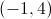and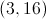. What is the midpoint of this segment?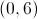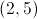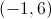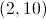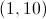Explanation:

To solve this problem you will need to use the midpoint formula: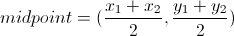Plug in the given values for the endpoints of the segment:and.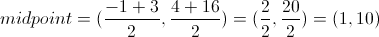### Example Question #6 : How To Find The Midpoint Of A Line Segment

What is the midpoint between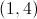and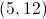?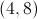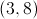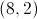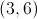Explanation:

The midpoint is the point halfway between the two endpoints, so sum up the coordinates and divide by 2: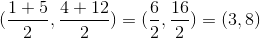← Previous 1

### All SAT Math Resources